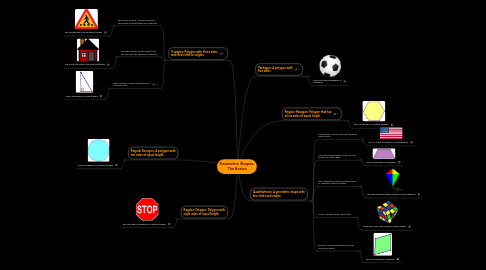# Geometric Shapes: The Basics

Get Started. It's FreeGeometric Shapes: The Basics## 3. Quadrilaterals: A geometric shape with four sides and angles.

### 3.1. Parallelogram: Quadrilateral with opposite sides parallel.

3.1.1. The U.S. flag is an example of a parallelogram.

### 3.2. Trapezoid: Quadrilateral that has at least one pair of parallel sides.

3.2.1. This is an example of a trapezoid.

### 3.3. Kite: Quadrilateral with two distinct pairs of congruent adjacent lengths.

3.3.1. The kites we played with as children is a great example.

### 3.4. Square: Rhombus with a right angle.

3.4.1. This Rubik's Cube is an example of many squares.

### 3.5. Rhombus: Quadrilateral where all sides have equal length.

3.5.1. This is an example of a rhombus.

## 4. Triangles: Polygon with three sides and three interior angles.

### 4.1. Equilaterial Triangle: Polygon in which all three sides and three angles are congruent.

4.1.1. This crossing sign is an equilateral triangle.

### 4.2. Isosceles Triangle: Polygon where only two sides and two angles are congruent.

4.2.1. The roof of this house is an isosceles triangle.

### 4.3. Right Triangle: Polygon that has exactly one right angle.

4.3.1. This is an example of a right triangle.